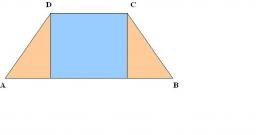# Calculate 3161

In the isosceles trapezoid ABCD, the arm is 5.2 cm long, the middle bar is 7 cm long, and the height is 4.8 cm. Calculate the lengths of both bases.

a =  9 cm
c =  5 cm

### Step-by-step explanation:Did you find an error or inaccuracy? Feel free to write us. Thank you!

Tips for related online calculators
Looking for help with calculating arithmetic mean?
Looking for a statistical calculator?
Do you have a linear equation or system of equations and looking for its solution? Or do you have a quadratic equation?
The Pythagorean theorem is the base for the right triangle calculator.
Calculation of an isosceles triangle.